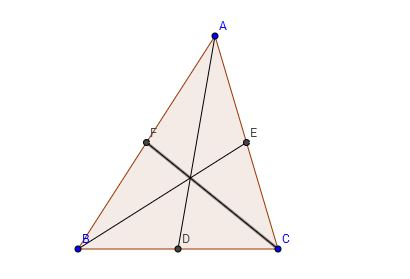Side Median Ratio

Geometry Level 3$AD$, $BE$ and $CF$ are the medians of the triangle $ABC$. If $AD \perp BE$, then $\dfrac{AB}{CF}$ can be expressed as $\dfrac{p}{q}$ where $p$ and $q$ are co-prime positive integers. What is $p+q$?

Details and assumptions: The picture isn't drawn accurately.

×